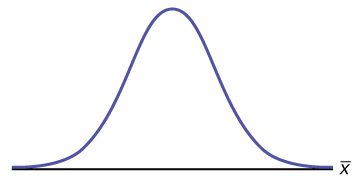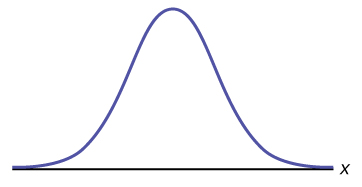# 7.1E: The Central Limit Theorem for Sample Means (Exercises)

•• OpenStax
• OpenStax
$$\newcommand{\vecs}{\overset { \rightharpoonup} {\mathbf{#1}} }$$ $$\newcommand{\vecd}{\overset{-\!-\!\rightharpoonup}{\vphantom{a}\smash {#1}}}$$$$\newcommand{\id}{\mathrm{id}}$$ $$\newcommand{\Span}{\mathrm{span}}$$ $$\newcommand{\kernel}{\mathrm{null}\,}$$ $$\newcommand{\range}{\mathrm{range}\,}$$ $$\newcommand{\RealPart}{\mathrm{Re}}$$ $$\newcommand{\ImaginaryPart}{\mathrm{Im}}$$ $$\newcommand{\Argument}{\mathrm{Arg}}$$ $$\newcommand{\norm}{\| #1 \|}$$ $$\newcommand{\inner}{\langle #1, #2 \rangle}$$ $$\newcommand{\Span}{\mathrm{span}}$$ $$\newcommand{\id}{\mathrm{id}}$$ $$\newcommand{\Span}{\mathrm{span}}$$ $$\newcommand{\kernel}{\mathrm{null}\,}$$ $$\newcommand{\range}{\mathrm{range}\,}$$ $$\newcommand{\RealPart}{\mathrm{Re}}$$ $$\newcommand{\ImaginaryPart}{\mathrm{Im}}$$ $$\newcommand{\Argument}{\mathrm{Arg}}$$ $$\newcommand{\norm}{\| #1 \|}$$ $$\newcommand{\inner}{\langle #1, #2 \rangle}$$ $$\newcommand{\Span}{\mathrm{span}}$$$$\newcommand{\AA}{\unicode[.8,0]{x212B}}$$

Use the following information to answer the next six exercises: Yoonie is a personnel manager in a large corporation. Each month she must review 16 of the employees. From past experience, she has found that the reviews take her approximately four hours each to do with a population standard deviation of 1.2 hours. Let $$X$$ be the random variable representing the time it takes her to complete one review. Assume $$X$$ is normally distributed. Let $$\bar{X}$$ be the random variable representing the mean time to complete the 16 reviews. Assume that the 16 reviews represent a random set of reviews.

##### Example $$\PageIndex{1}$$

What is the mean, standard deviation, and sample size?

mean = 4 hours; standard deviation = 1.2 hours; sample size = 16

Exercise $$\PageIndex{2}$$

Complete the distributions.

1. $$X \sim$$ _____(_____,_____)
2. $$\bar{X} \sim$$ _____(_____,_____)
##### Example $$\PageIndex{3}$$

Find the probability that one review will take Yoonie from 3.5 to 4.25 hours. Sketch the graph, labeling and scaling the horizontal axis. Shade the region corresponding to the probability.Figure $$\PageIndex{2}$$.

2. $$P$$(________ $$< x <$$ ________) = _______

1. Check student's solution.
2. 3.5, 4.25, 0.2441

Exercise $$\PageIndex{4}$$

Find the probability that the mean of a month’s reviews will take Yoonie from 3.5 to 4.25 hrs. Sketch the graph, labeling and scaling the horizontal axis. Shade the region corresponding to the probability.Figure $$\PageIndex{3}$$.

2. $$P$$(________________) = _______

##### Example $$\PageIndex{5}$$

What causes the probabilities in Exercise and Exercise to be different?

The fact that the two distributions are different accounts for the different probabilities.

Exercise $$\PageIndex{6}$$

Find the 95th percentile for the mean time to complete one month's reviews. Sketch the graph.Figure $$\PageIndex{4}$$.

1. The 95th Percentile =____________

This page titled 7.1E: The Central Limit Theorem for Sample Means (Exercises) is shared under a CC BY 4.0 license and was authored, remixed, and/or curated by OpenStax via source content that was edited to the style and standards of the LibreTexts platform; a detailed edit history is available upon request.